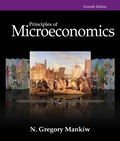# Raj opens up a lemonade stand for two hours He spends $10 for ingredients and sells$60 worth of lemonade. In the same two hours, he could have mowed his neighbor’s lawn for $40. Raj has an accounting profit of _____ and an economic profit of _____. a.$50, $10 b.$90, $50 c.$10, $50 d.$50, $90BuyFind ### Principles of Microeconomics 7th Edition N. Gregory Mankiw Publisher: Cengage Learning ISBN: 9781305156050BuyFind ### Principles of Microeconomics 7th Edition N. Gregory Mankiw Publisher: Cengage Learning ISBN: 9781305156050 #### Solutions Chapter Section Chapter 13, Problem 1CQQ Textbook Problem ## Raj opens up a lemonade stand for two hours He spends$10 for ingredients and sells $60 worth of lemonade. In the same two hours, he could have mowed his neighbor’s lawn for$40. Raj has an accounting profit of _____ and an economic profit of _____. a. $50,$10 b. $90,$50 c. $10,$50 d. $50,$90

Expert Solution
To determine
Accounting profit and economic profit.

Option ‘a’ is correct.

### Explanation of Solution

Option (a):

When Raj spend $10 for ingredients and sell$60 worth of lemonade, then the accounting profit will be (Total revenueTotal explicit cost) (total revenue – total explicit cost) $50($60$10). Economic profit is (Total revenue(Implicit cost +Explicit cost))$10 ($60($50+$10)) . Therefore, the value of accounting profit is$50 and the economic profit is $10. Option (b): Raj accounting profit is not$90, because an accounting profit is the total revenue minus the total explicit cost. Economic profit is not $50, because economic profit is total revenue minus implicit and explicit cost. Thus, option ‘b’ is incorrect. Option (c): When Raj spends$10 for ingredients and sells $60 worth of lemonade, Raj accounting profit will not be$10, because an accounting profit is the total revenue minus the total explicit cost. Economic profit is not $50, because economic profit is the total revenue minus implicit and explicit cost. Thus, option ‘c’ is incorrect. Option (d): When Raj spend$10 for ingredients and sell $60 worth of lemonade, then the accounting profit will be (total revenue – total explicit cost)$50($60$10) . But the economic profit is not \$90, because the economic profit is (Total revenue(Implicit cost +Explicit cost)) . Thus, option ‘d’ is incorrect.

Economics Concept Introduction

Concept introduction:

Accounting profit: Accounting profit refers to the total revenue minus total explicit cost

Economic profit: Economic profit refers to the total revenue minus implicit and explicit cost.

### Want to see more full solutions like this?

Subscribe now to access step-by-step solutions to millions of textbook problems written by subject matter experts!

Get Solutions

### Want to see more full solutions like this?

Subscribe now to access step-by-step solutions to millions of textbook problems written by subject matter experts!

Get Solutions

Find more solutions based on key concepts
Show solutions
What is the difference between a cash discount and a trade discount?

College Accounting (Book Only): A Career Approach

REGRESSION AND RECEIVABLES Edwards Industries has 320 million in sales. The company expects that its sales will...

Fundamentals of Financial Management, Concise Edition (with Thomson ONE - Business School Edition, 1 term (6 months) Printed Access Card) (MindTap Course List)

When using normal costing, how are jobs charged with overhead?

Cornerstones of Cost Management (Cornerstones Series)